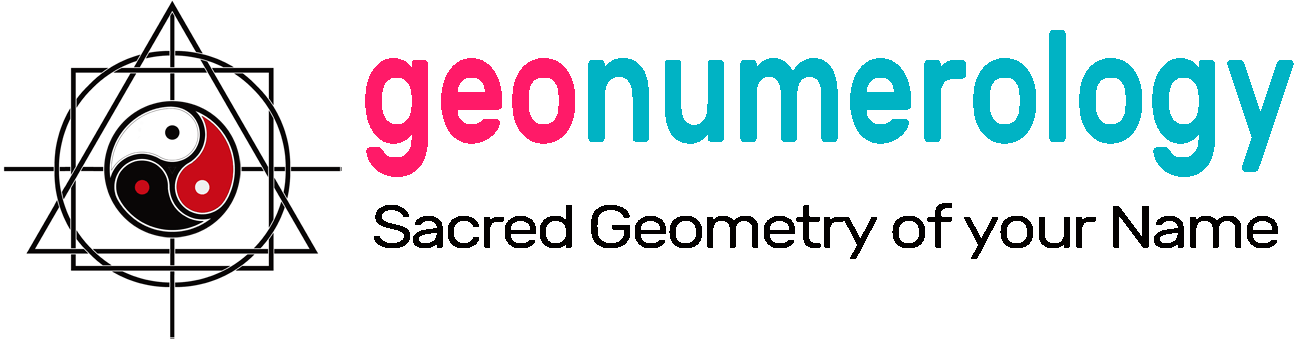The First Personality Reading Based On
The Sacred Geometry Of Your Name Symbols.

# Numerology Charts

Current numerology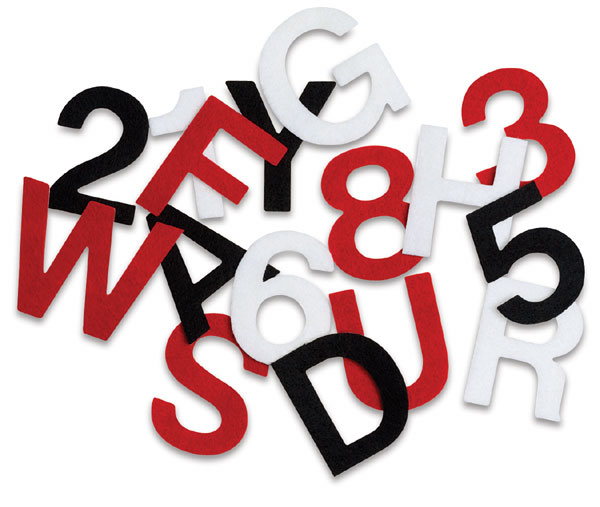## Analogy and correspondences

The number and its representations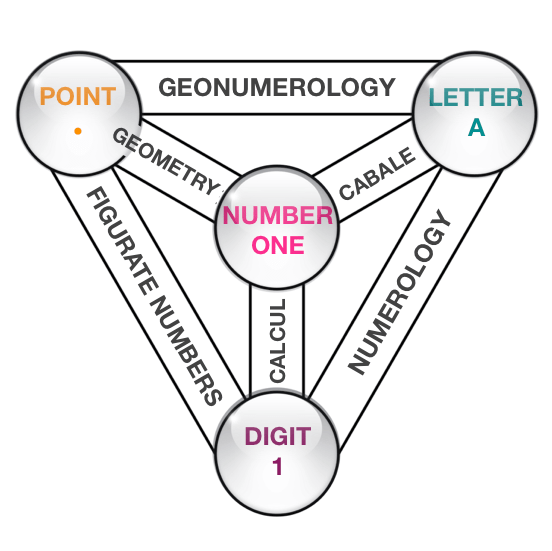### Law of Analogy

#### ONE = •

Man learned to count long before he could write. Using notches in bones or wood and then small pebbles, from the Latin calculi, plural of calculus.

#### ONE = A

Then with the arrival of writing, letters were then used to designate numbers. This is notably the case of the Hebrew and Greek alphabets. The number 1 is thus associated with the letter Alpha in Greek or Aleph in Hebrew.

#### ONE = 1

The graphisme of the numbers (1,2,3, ...) that we use for the calculation, is of Indian origin and was transmitted to Western civilisation by the Arabs in the 10th century.

### Theory of correspondences

#### If A = 1

Each letter has a numerical value

➣ This is principle of Numerology.

#### And 1 = •

To the arithmetic unit unit corresponds the geometrical point.

➣ This is the principle of geometry.

#### So A = •

It is therefore possible to to visualize any word or Name according to the number of units it contains.

➣ Geonumerology synthesis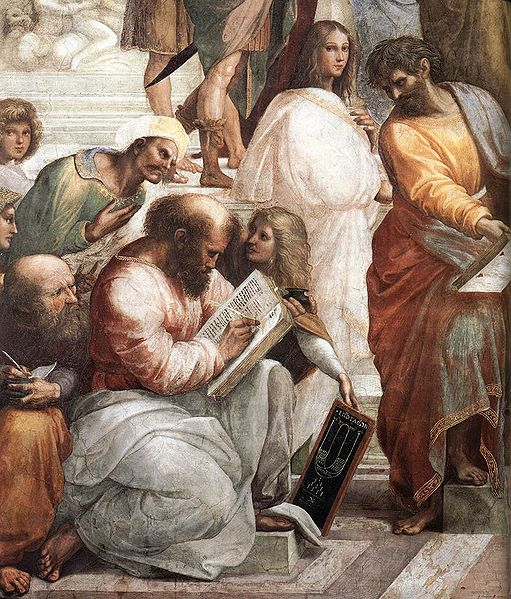### Pythagoras ... and copiers

« We must not confuse Numbers, which are Ideas-Strengths, intermediaries between the Visible Plane and the Invisible Plane, and Digits, which are the clothing of numbers. »

The Science of Numbers
by Papus (Dr Gérard Encausse) - 1934
Posthumous work

## The principle of Reduction

Philosophical calcul

#### Reduction of numbers from 10 to 18In the table above we see that the series of the first nine numbers appears again with the number 10 and ends with the number 18.
The next series starts with 19 = 1 + 9 = 10 = 1 and ends with 27 = 2 + 7 = 9 and so on.

The theosophical reduction (from the Greek: theos, divine and sophia, wisdom) corresponds to the current 9-number numerology.

It consists in reducing all numbers made up of two or more digits to single-digit numbers by adding up the digits that make up the number until there is only one left.

Example: 58 = 5 + 8 = 13
and 13 = 1 + 3 = 4

« The theosophical reduction was an operation familiar to Pythagoreans who neglected numbers greater than 10,
so they reduced numbers greater than 10 to the first nine numbers, taking into account only their root or pythmen, i.e., substituting the remainder of their division by nine, or the number nine even
when the number was a multiple of nine. »

Arthuro Reghini ~
Sacred Numbers in the Pythagorean Masonic Tradition

## Analogy between letters and numbers

Principle of numerology

#### The number of your name

Numerology takes up the teachings of Pythagoras and associates a numerical value to each letter: 1 for A, 2 for B, 3 for C, etc.

For numbers with more than one digit, we apply theosophical reduction which adds up the digits of the number so that only one remains. Ex: J = 10 = 1 + 0 = 1, K = 11 = 1 = 1 = 2, etc.

Thus it is possible to calculate the Number of your Name, by adding up the letters in it, and then, by reducing the total, to find a number between 1 and 9.

« The Pythagoreans assured that each letter has a certain number which indicates the future; that one can guess what must happen to men by calculating the numbers contained in the letters of their proper names. »

Agrippa - De Vanitate Scientiarum

### Correspondences between letters and numbers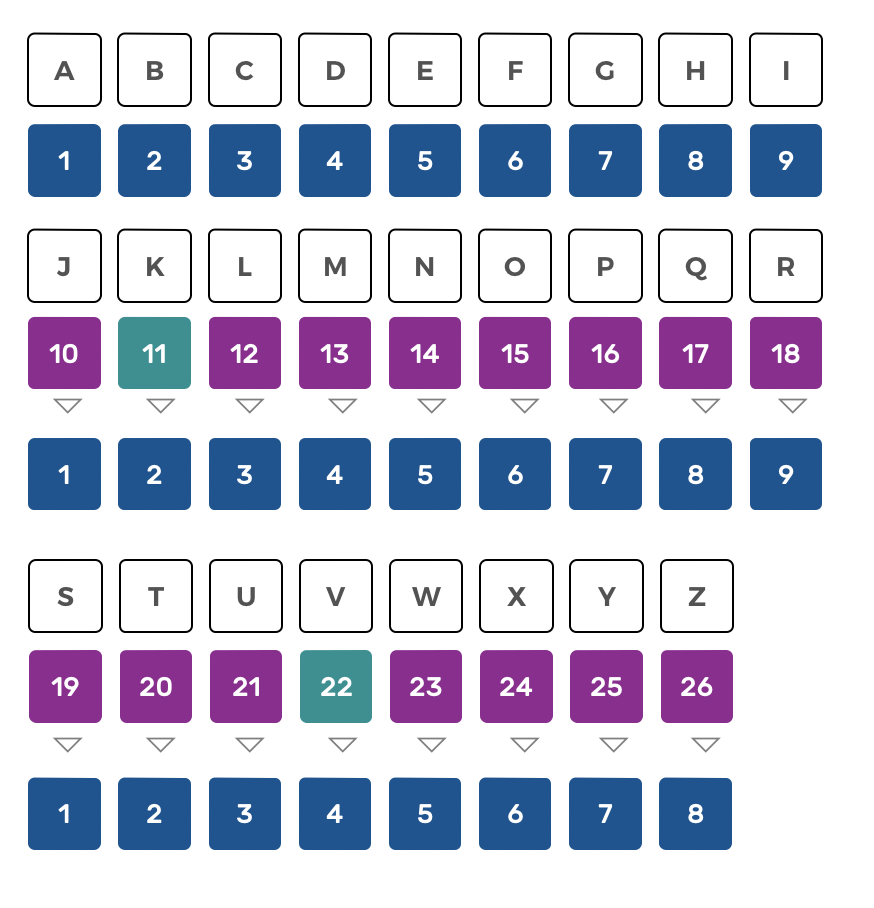Contemporary numerology does not practice reduction for the numbers 11 and 22, considering them as master numbers. We will see below whether this makes sense or not.

## Alpha-numeric tableWrite your LAST NAME and FIRST NAME in capital letters and then indicate just below the value of each letter, using the mnemonic matrix.

Then add up the total until you get a number between 1 and 9. Remember to memorize the last operation that precedes the reduced number. For example 4 obtained by 1 + 3, 2 + 2 or 4 + 0.

Man receives a name at birth, which will distinguish him from others but above all characterizes his Personal Vibratory Signature. This vibration varies for each being according to the frequency and, evolves in time. To know the name of an individual, of a thing, is to know his being. The Name has therefore hugely importance, because by its vibrations it expresses the essence of a being or an object.

## Master numbers or selfish numbers

Let's have a look inside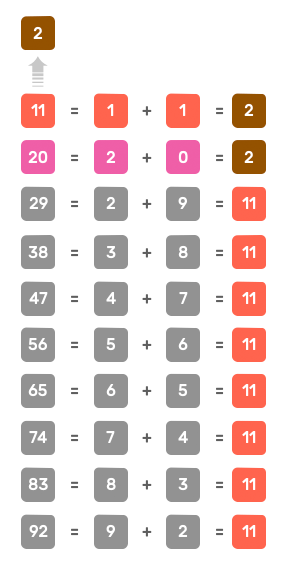Of the ten decompositions identified above, only one involves the number 20 and nine hugely the number 11, i.e. 90% of the cases.
In other words,
all the two are eleven !

#### Master number 11

Modern numerology distinguishes two cases of what it calls ‘Master Numbers’ : 11 and 22, which are not subjected to reduction. Pythagoras considered these dual numbers to be egotistic, as they were formed from a single number that is paired with itself.

Additionally, this designation as ‘Master Numbers’ seems largely exaggerated, considering that the number 2 is obtained 90% of the time from 11 (29, 38, 47, 56, 65, 74, 83, 92, etc.). The only one that is not created in this way is the 2 obtained from 20: 2 + 0 = 2 (see the table on the left).

>> Source-Number 11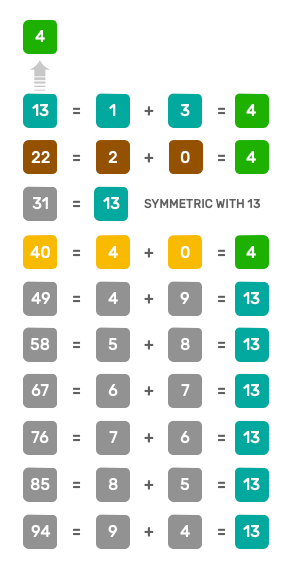Of the ten decompositions identified above, only one involves the number 22 and one number 40, while seven involve the number13, i.e. 70% of the cases.
In other words the number 4 is not always a square

#### Master number 22

The number 4 can only be obtained in three ways:

• 1 + 3 = 4
• 2 + 2 = 4
• 4 + 0 = 4

Decomposition 2 + 2 is as rare as decomposition 4 + 0.

Finally the number 13 is the most common symbol for number 4.

In Geonumerology, importance is placed on the decomposition of all the numbers preceding the 9 reduced numbers. There are only 29 in all. These are the "Source-Numbers" of Geonumerology.

>> Source-Number 22

## Numerology current interpretation

Very far from the Pythagorean holistic vision

Current numerology relates numbers to ideas in a way that often remains unknown to the reader and depends largely on the interpretation of each author. More often than not, we find ourselves faced with a linear interpretation: one number, then another number, etc.

The number is interpreted without the slightest reference to the structure used to accommodate the numbers or to the links between them.

There are also numerologists who base their interpretations on the graphisme of the digits that are supposed to represent numbers, thus confusing the number with its representative.

Papus, in The Science of Numbers, warns us against making two mistakes:

« The first one is to distinguish the quantitative study of numbers as pursued by current mathematicians
from the qualitative study as it was pursued in the initiation centers of antiquity.

The second distinction to make is not to confuse the Numbers which are beings, with the Numbers which are their clothing. A man dressed in green, yellow or black is always himself. A number represented by a Chinese, or Sanskrit, or Roman, or current typographic character is always himself. But the question number has often confused the question number. »

Numbers
Persona
Verb
Keyword
Quality
Number 1
Politician
Dominate
Aspirations
New
Volunteer
Number 2
Psychologist
Reflect
Relationship
Balanced
Sensitive
Number 3
Artist
Create
Original
Communication
Passionate
Number 4
Architect
Organize
Effective
Logical
Reasoning
Number 5
Choose
Bold
Free
Animation
Number 6
Therapist
Harmonizing
Artistic
Humanistic
Responsibilities
Number 7
Researcher
Reaching out
Spiritual
Perfectionist
Studies
Number 8
Company manager
Construction
Materialization
Material
Responsible
Number 9
Philosopher
Sharing
Altruistic
Universal
Vocation

The table above shows the main characteristics attributed to the first 9 numbers. Would you be surprised if I told you that all 4 are not organized and that all 9 are not altruistic.

## Numerology Defects

A monolithic and ungrounded linear vision

The main difference between numerology and geo-numerology, in addition to its graphical approach, is the adoption of a systemic approach where the relationships between numbers are more important than an isolated interpretation.

Geonumerology links psychology to spirituality without succumbing to the pitfalls of mysticism. Its method is rational and logical because it is based on the rules of arithmetic and sacred geometry, a true criterion of truth.

A notable difference between the two disciplines lies in the fact that numerology interpretation is most often isolated: one number, then another number, whereas the systemic approach used in GeoNumerology is closer to the holistic vision of Pythagoras than to a Cartesian analytical vision.

As the name suggests, it is a question of studying a system. A system is composed of a certain number of elements (here the 9 numbers), arranged within a structure (here a matrix of 9 boxes) and establishing relationships between them (which we will explain).

The main tools of systems thinking such as analogical reasoning, isomorphism, graphic language or modeling are strictly the same as those used in GeoNumerology.

The interpretation of most numerologists comes from what I would call "ungrounded" reasoning, without the slightest reference to the structure used to accommodate the first 9 numbers, nor to the links between these 9 numbers.

Most of the available numerical interpretations work like this:

• if your expression number is x, you're like this;
• if your soul urge number is y, you are like this.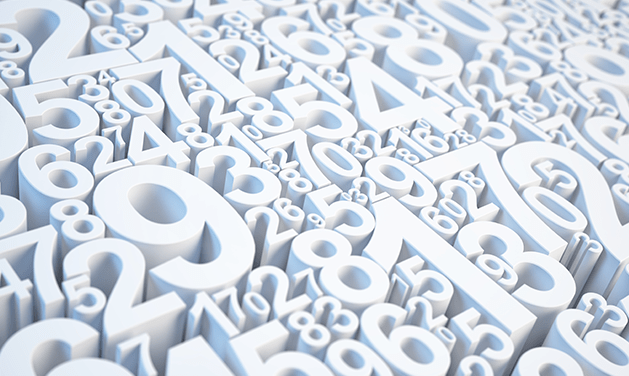We have just seen that the number 4 had three different representations One cannot reasonably interpret in the same way a 4 resulting from 1 + 3, 2 + 2 ou 4 + 0, knowing that the first represents a tetrahedron, the second a cross and the third a square.

The 29 decompositions that precede the 9 reduced numbers are the arithmetic keys that will allow us to do two things :

• Visualize a number in geometric space by adding up one by one the units that comprise it;
• Locate a number and its components in the numeric matrix of the Three Worlds, revealing its role in relation to the whole.

## From Numerology to Geonumerology

Back to the sources of the true numerology of Pythagoras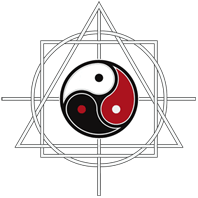Geonumerology is an interdisciplinary approach that aims to restore the ancient numerology of Pythagoras.

Etymologically, one could say that numerology is a "discourse on numbers" whereas geonumerology is the science of the link (Logos) that exists between numbers (Numero) and geometry (Geo). In this way, it allows the number to be represented, to make it visible in space, to give it shape. It is only after having drawn it that the interpretation of the number takes all its meaning and begins to reveal its secrets.THE SACRED GEOMETRY OF YOUR NAME